Main Module 3 Multiple Regression and Model Building

 Learning Objectives At the end of the module, the student will: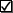Know that multiple regression and correlation concerns the relationship (form, direction and strength) between a dependent variable and multiple independent variables.Understand how to present, test, describe and interpret : the relationship between a dependent variable and multiple independent quantitative variables; and use relationship for prediction. the relationship between a dependent variable and qualitative independent variables; and use the relationship for prediction.   curvature and interaction; and use curvature and interaction models for prediction.Know how to hypothesize, build and use for prediction, multiple regression models with possible significant quantitative, qualitative, curvature and interaction terms.Be able to use Microsoft Excel for the multiple regression and correlation analysis.
 Module Notes The following sub modules contain summary notes for the three content topic areas of Module 3.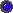Module 3.1: Present, describe and test relationship between response and multiple explanatory variables.Module 3.2: Present, describe and test relationships involving interaction, curvature, and dummy independent variables.Module 3.3: Multiple regression model building process. Assignment Select a realistic and interesting data set consisting of a sample of approximately 50 observations (n = 50). Your data set should include a quantitative dependent variable (Y), and two independent (X - predictor) variables, one quantitative (QN) and one qualitative (QL). Example: Y = Salary of USF Professor QN = Years of Experience QL = Gender ( 1 = Male; 0 = Female) Please select a qualitative variable with exactly two-levels (male/female; in-season/off-season; large cap fund/small cap fund). Also, try to select about the same number of data points for each level, for example, 25 males and 25 females. 1. In an Excel Spreadsheet, enter the QN data in a column, create a column for curvature (QN^2, where "^" represents "squared") data, enter the QL data in a column, create a column for QN*QL interaction (where "*" indicates multiple respective QN data times QL data, and enter Y data in a column. Note: all independent variables should be contiguous or adjacent to each other). 2. Using the data analysis tool, build and test at least two of the following models, in the process of determining your "best" model. For each test, be able to state the null and alternate hypotheses, the hypothesized regression equation associated with the null and alternate hypotheses, the appropriate p-value, the null hypothesis decision (reject or do not reject the null hypothesis), and the resulting conclusion (e.g., curvature is important, interaction is not important or not significant). A flowchart to guide model testing is provided in Item 4. Model 1: E(Y) = B0 + B1 QN + B2 QN2 + B3 QL + B4 QN*QL Model 2: E(Y) = B0 + B1 QN + B3 QL + B4 QN*QL Model 3: E(Y) = B0 + B1 QN + B2 QN2 + B3 QL Model 4: E(Y) = B0 + B1 QN + B2 QN2 Model 5: E(Y) = B0 + B1 QN + B3 QL Model 6: E(Y) = B0 + B3 QL Model 7: E(Y) = B0 + B1 QN 3. Using the data analysis regression tool, build Model 1. 4. Test curvature (Model 1 vs. 2). A. If curvature is significant, test interaction (Model 1 vs. 3). (1). If interaction is significant, stop. Model 1 is "best" model. Go to Item 5. (2). If interaction is not significant, Build Model 3 and test QL (Model 3 vs. 4). a. If QL is significant, stop. Model 3 is "best" model. Go to Item 5. b. If QL is not significant, build Model 4 and stop. Model 4 is "best" model. Go to Item 5. B. If curvature is not significant, build Model 2 and test interaction (Model 2 vs. 5). (1). If interaction is significant, stop. Model 2 is "best" model. Go to item 5. (2). If interaction is not significant, build Model 5 and test QL (Model 5 vs. 7). a. If QL is significant, test QN (Model 5 vs. 6). 1. If QN is significant, stop. Model 5 is "best" model. Go to Item 5. 2. If QN is not significant, stop. Model 6 is "best model. Go to Item 5. b. If QL is not significant, stop and select Model 7 as "best" model, even if the Model is not significant. Go to Item 5. 5. Rerun the data analysis regression tool for your "best" model, and include and be able to describe or interpret the following printouts: Residual plot : QN (Model 4 or 7) QL Model 6) QN and QL (Models 1, 2, 3, or 5) Normal probability plot (for all Models) Fitted Line Plot: QN2 (Models 1, 3, 4) QN (Models 1, 2, 3, 4, 5, 7) QL (Models 1, 2, 3, 5, 6) 6. Be able to demonstrate your knowledge of the learning objectives as applied to this Assignment in Exam 3. 7. (Optional) Send Microsoft Excel file, with Items 1 - 5, as an attachment in an e-mail to the instructor if you wish the instructor to review/give feedback on your work. This should be done not later than November 1, 2001 if you wish to receive instructor feedback. You may send parts of the assignment as you finish them, if you wish. 8. Notify the instructor, via e-mail, when you have completed Assignment 3, and wish to take Exam 3. Include Assignment 3 as an attachment to your e-mail if you did not do item 7 above. This needs to be done BEFORE Nov 3, 2001. E-mail or fax completed Exam 3 back to the instructor NOT LATER THAN NOV 3, 2001. Optional Text Reading Anderson, D., Sweeney, D., & Williams, T. (2001). Contemporary Business Statistics with Microsoft Excel. Cincinnati, OH: South-Western. Chapter 12 (Section 12.9).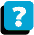About the Course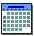Module Schedule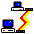WebBoard